﻿ 基于改进粒子群算法的移动机器人多目标点路径规划
«上一篇文章快速检索 高级检索

 智能系统学报2017, Vol. 12Issue (3): 301-309  DOI: 10.11992/tis.2016060460

引用本文PU Xingcheng, LI Junjie, WU Huichao, et al. Mobile robot multi-goal path planning using improved particle swarm optimization[J]. CAAI Transactions on Intelligent Systems, 2017, 12(3): 301-309. DOI: 10.11992/tis.201606046.文章历史

1. 重庆邮电大学 数理学院, 重庆 400065;
2. 重庆邮电大学 智能系统及机器人研究所, 重庆 400065;
3. 重庆邮电大学 先进制造学院, 重庆 400065

Mobile robot multi-goal path planning using improved particle swarm optimization
PU Xingcheng1, LI Junjie2, WU Huichao2, ZHANG Yi3
1. School of Science, Chongqing University of Posts and Telecommunications, Chongqing 400065, China;
2. Research Center of Intelligent System and Robot, Chongqing University of Posts and Telecommunications, Chongqing 400065, China;
3. Advanced Manufacturing Engineering School, Chongqing University of Posts and Telecommunications, Chongqing 400065, China
Abstract: To solve the problem of multi-goal path planning for mobile robots that pass multiple goals, a new path planning method, based on improved particle swarm optimization (PSO) and ant colony optimization (ACO), is proposed. In this new method, the first step is to use an improved PSO, which has high convergence, to optimize the path between two goals among a sequence of goals. The second step is to use the ACO to obtain the shortest path for all target points. The performance experimental result demonstrates that the improved PSO algorithm can effectively avoid premature convergence and enhances search ability and stability. Simulation results show that the improved PSO algorithm can make a mobile robot realize collision-free multi-goal path planning effectively. Experiments in a real environment demonstrate that this algorithm has practical application for multi-goal path planning.
Key words: mobile robot    multi-goal path planning    ACO    improved PSO    opposition-based learning    inertia weight    learning factors

1 问题描述 1.1 多目标点路径规划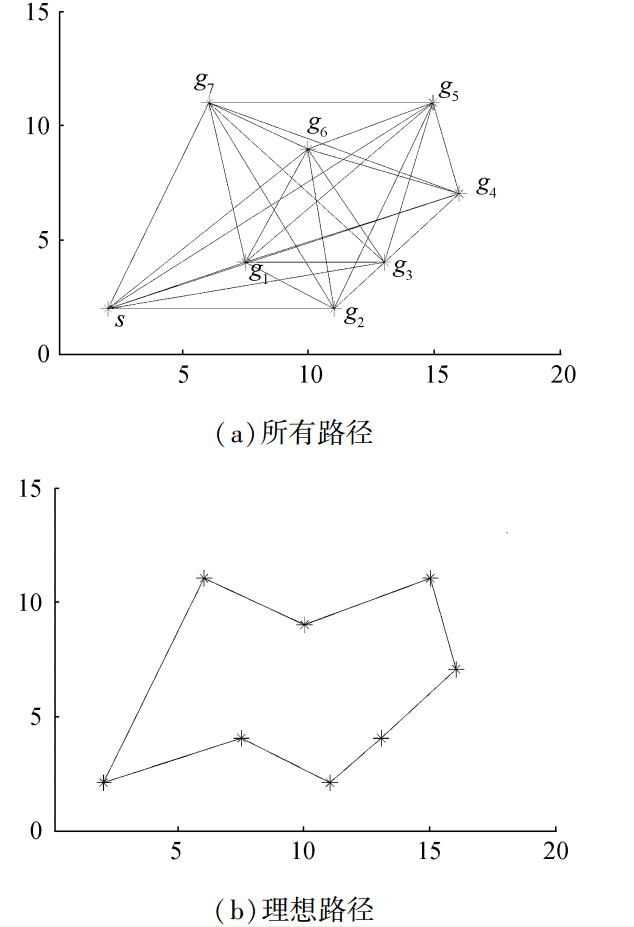图 1 多点之间的路径轨迹 Fig.1 The path trajectory of multi-goal

1.2 粒子的适应度函数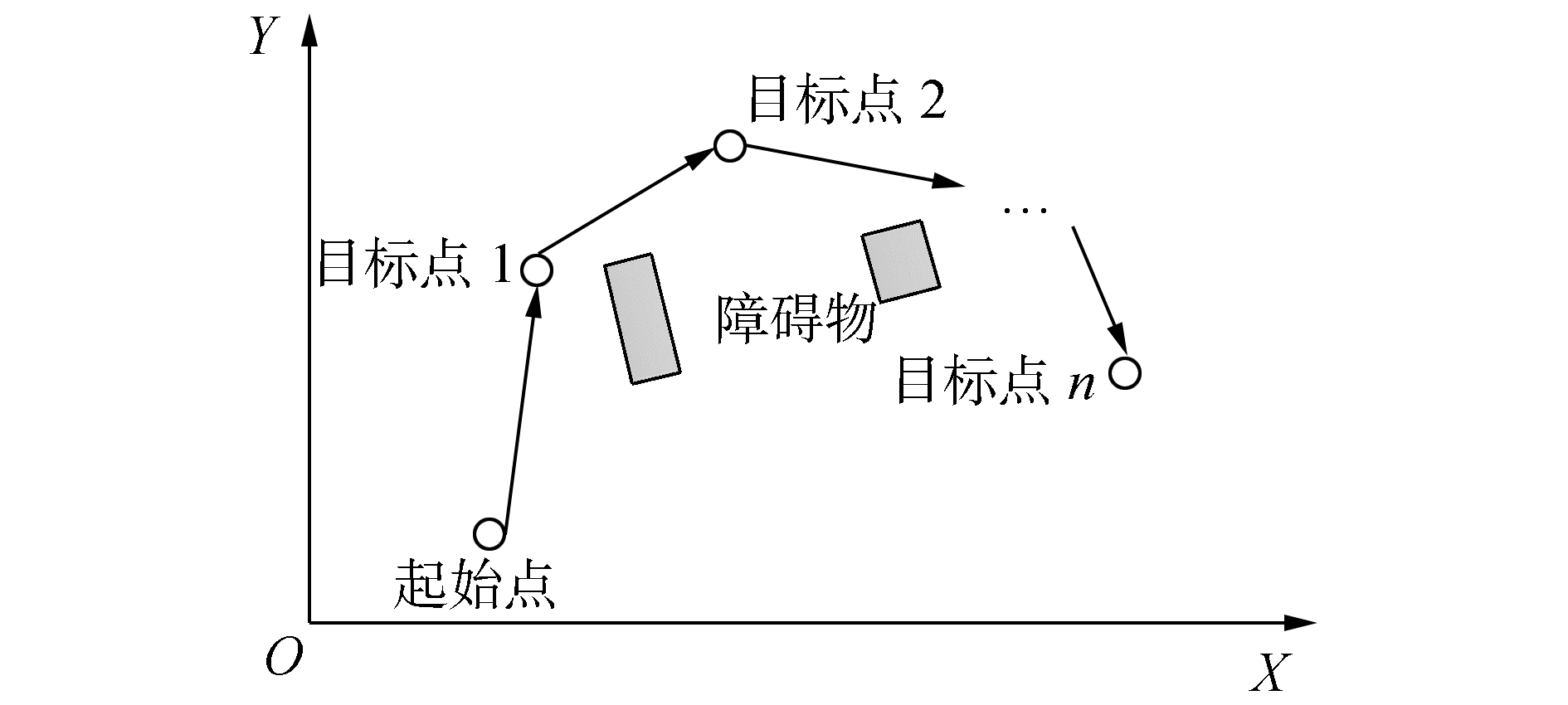图 2 移动机器人多目标点路径规划示意图 Fig.2 The diagram of mobile robot multi-goal path planning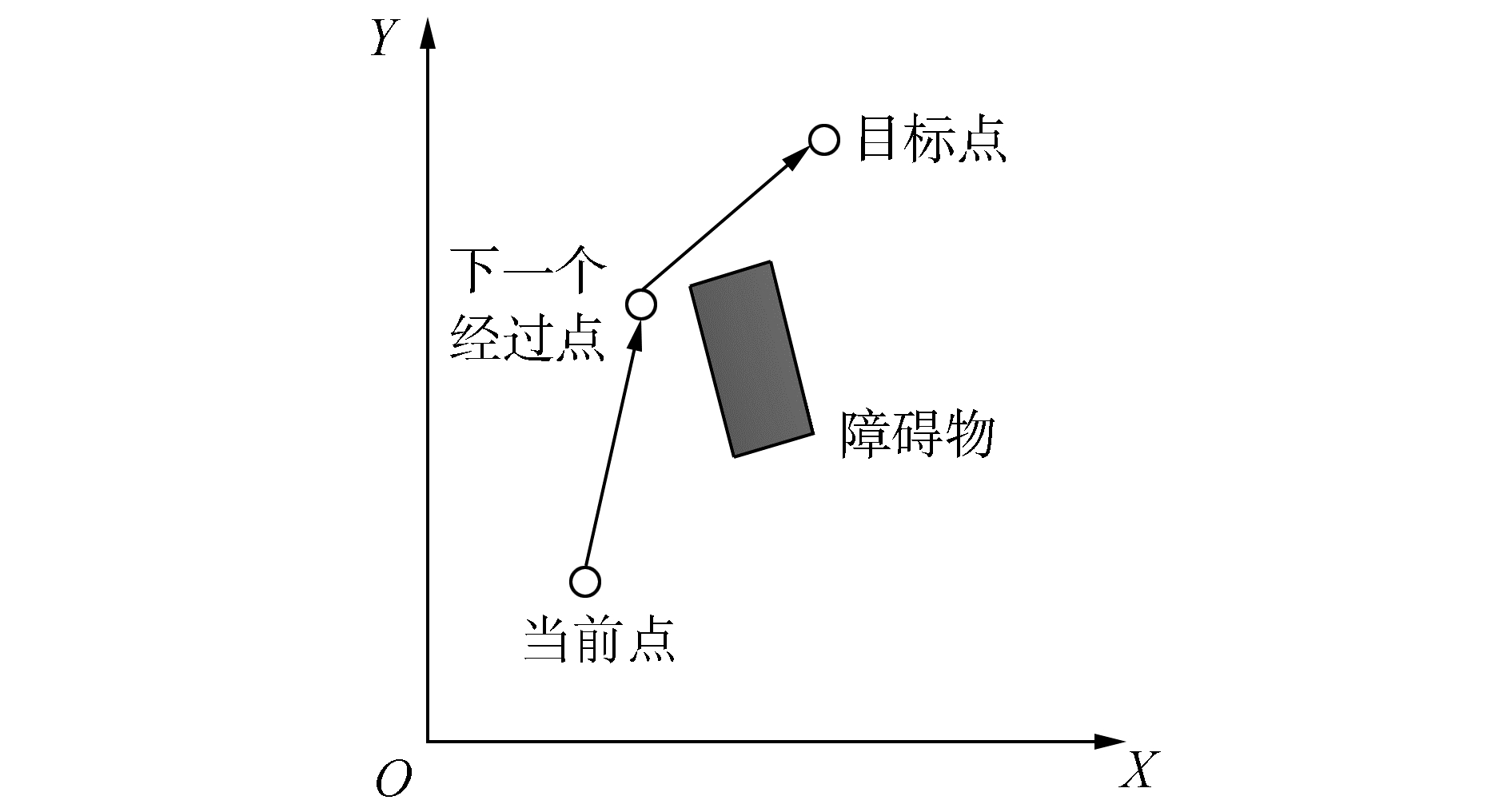图 3 当前点到目标点避障描述 Fig.3 The obstacle avoidance description of currentpoint to goal

 $\begin{array}{l} {F_1} = \sum\limits_{j = 1}^g {\sum\limits_{i = 1}^S {\left[{\sqrt {{{\left( {x_{i, j}^{{\rm{current}}}-x_{i, j}^{{\rm{next}}}} \right)}^2} + {{\left( {y_{i, j}^{{\rm{current}}}-y_{i, j}^{{\rm{next}}}} \right)}^2}} + } \right.} } \\ \;\;\;\;\;\;\;\;\left. {\sqrt {{{\left( {x_{i, j}^{{\rm{next}}}-x_{i, j}^{{\rm{goal}}}} \right)}^2} + {{\left( {y_{i, j}^{{\rm{next}}} - y_{i, j}^{{\rm{goal}}}} \right)}^2}} } \right] \end{array}$

 ${F_2} = \left\{ \begin{array}{l} {k_r} \times {\left( {\frac{1}{{d\left( {{x_R}, {x_O}} \right)}}-\frac{1}{{{d_m}}}} \right)^2}{d^2}\left( {{x_R}, {x_G}} \right), \;\;\;d\left( {{x_{R, }}{x_0}} \right) \le {d_m}\\ 0, \;\;\;\;\;\;\;d\left( {{x_{R, }}{x_0}} \right) > {d_m} \end{array} \right.$

 $F = \lambda {F_1} + \mu {F_2}$

2 基本知识 2.1 标准粒子群算法

 $\mathit{\boldsymbol{v}}_i^{t + 1} = \omega \mathit{\boldsymbol{v}}_i^t + {c_1}{r_1}\left( {{\mathit{\boldsymbol{p}}_{{\rm{best}}}}-\mathit{\boldsymbol{x}}_i^t} \right) + {c_2}{r_2}\left( {{\mathit{\boldsymbol{g}}_{{\rm{best}}}}-\mathit{\boldsymbol{x}}_i^t} \right)$ (1)
 $\mathit{\boldsymbol{x}}_i^{t + 1} = \mathit{\boldsymbol{x}}_i^t + \mathit{\boldsymbol{v}}_i^t$ (2)

2.2 反向学习策略

Tizhoosh在2005年基于相反数或对立点的概念提出了反向学习策略。在随后的发展中，该方法被应用于解决一些优化问题中[12-13]。反向坐标定义如下。

 $\tilde x = a + b-x$

 ${{\tilde x}_i} = {a_i} + {b_i}-{x_i}$

3 反向学习粒子群算法

3.1 初始化种群

3.2 迭代进化过程

 $\omega = {\omega _{\max }}-\left( {{\omega _{\max }}-{\omega _{\min }}} \right)\left( {1-\frac{{{\rm{dis}}{{\rm{t}}_i}}}{{\max \_{\rm{dist}}}}} \right)$

 $\begin{array}{l} {\rm{dis}}{{\rm{t}}_i} = {\left( {\sum\limits_{d = 1}^D {{\mathit{\boldsymbol{g}}_{{\rm{bes}}{{\rm{t}}_d}}}-{\mathit{\boldsymbol{x}}_{i, d}}} } \right)^{\frac{1}{2}}}\\ \max \_{\rm{dist}} = \mathop {\arg \max \left( {{\rm{dis}}{{\rm{t}}_i}} \right)}\limits_i \end{array}$

 ${c_1} = {c_{1\max }} \times {\left( {\frac{{{c_{1\min }}}}{{{c_{1\max }}}}} \right)^{\left( {\frac{t}{{{T_{\max }}}}} \right)}}$
 ${c_2} = {c_{2\min }} \times {\left( {\frac{{{c_{2\max }}}}{{{c_{2\min }}}}} \right)^{\left( {\frac{t}{{{T_{\max }}}}} \right)}}$

3.3 算法实现流程

1) 首先初始化种群P(S)，包括粒子的位置xi和速度vii=1, 2, …, SS是种群的大小，初始化惯性权重ω以及学习因子c1c2等参数。

2) 在速度和位置规定的范围内根据式(1)、(2) 更新第i个粒子的速度vi和位置xi

3) 计算第i个粒子的适应度值fi

4)ai=min(xi)。

5)bi=max(xi)。

6) 计算第i个粒子的位置和速度反向解：${{\mathit{\boldsymbol{\tilde x}}}_i} = {a_i} + {b_i}-{\mathit{\boldsymbol{x}}_i}$

7) 计算第i个粒子反向解的适应度值${{\tilde f}_i}$

8) 比较fi${{\tilde f}_i}$的大小，如果${{\tilde f}_i} < {f_i}$, 则${x_i} = {{\mathit{\boldsymbol{\tilde x}}}_i}, {f_i} = {{\tilde f}_i}$

9) 从最优适应度值中选出S个适应度值最好的粒子组成初始化种群。

10) 接下来同粒子群算法基本流程。

11) 算法满足结束条件，结束算法过程。

4 实验结果与分析 4.1 OBLIPSO性能测试表 1 测试函数参数设置 Tab.1 The parameter setting of test function

OBLIPSO算法参数设置为：粒子群大小为40，空间维度为2，ω=[0.4, 0.9]，c1=[1.25, 2.75]，c2=[0.5, 2.25]，最大迭代次数为100。表 2 平均最优值结果对比 Tab.2 The comparison of average best values表 3 标准方差结果对比 Tab.3 The comparison of standard deviation

4.2 路径规划仿真实验

1) 第1次实验中，OBLIPSO算法的参数设置为：ωmax=0.9，ωmin=0.4，S=10，c1max=2.75，c2max=2.25, c1min=1.25, c2min=0.5，vmax=1.9，vmin=0，斥力场中的安全距离dm=2，kr=2.7，λ=0.25，μ=0.25，最大迭代次数为100。图 4(a)给出了在简单环境下的OBLIPSO作用的移动机器人多目标点移动轨迹。图 4(b)是IAPSO作用的运动轨迹。图 4(c)是IPSO作用的运动轨迹。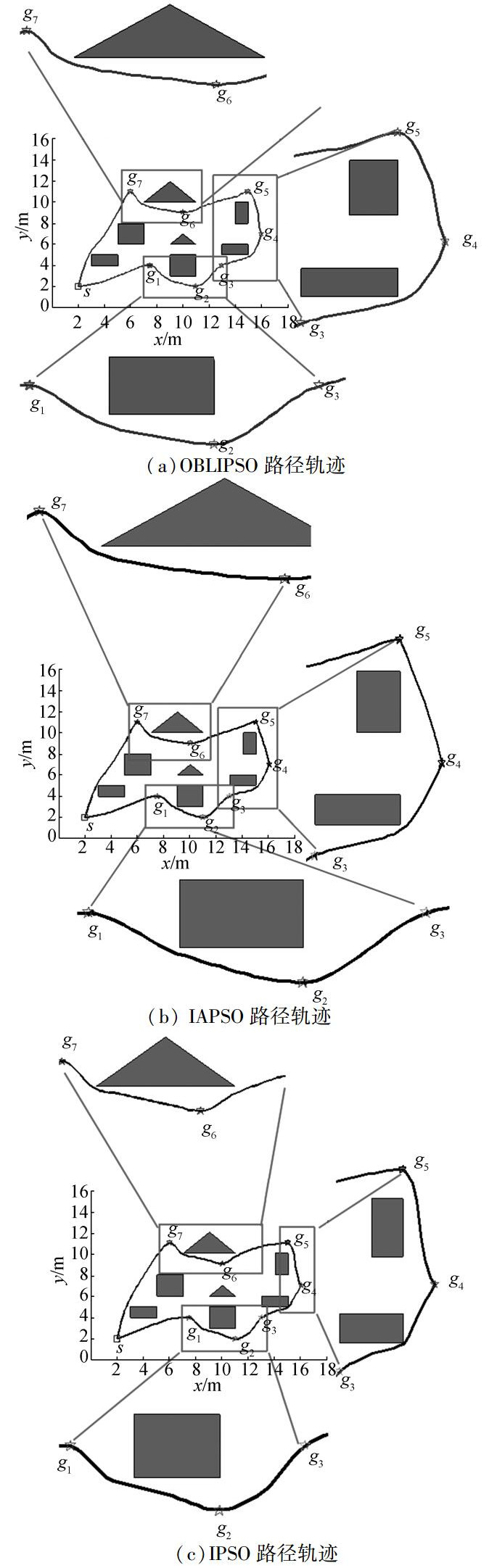图 4 简单环境下的优化过程 Fig.4 The motion process in the first environment

2) 在第2次实验中，实验环境相对第1次实验而言，增加了障碍物以及目标点的个数。实验参数设置如下：vmax=20，最大迭代次数为300，其余参数与第1次实验中参数相同。由于环境复杂度的增加，相对第1次实验而言，粒子收敛速度变慢。图 5(a)是在复杂环境下的OBLIPSO获得的移动机器人多目标点运动轨迹。图 5(b)是IAPSO获得的运动轨迹。图 5(c)是IPSO作用的运动轨迹。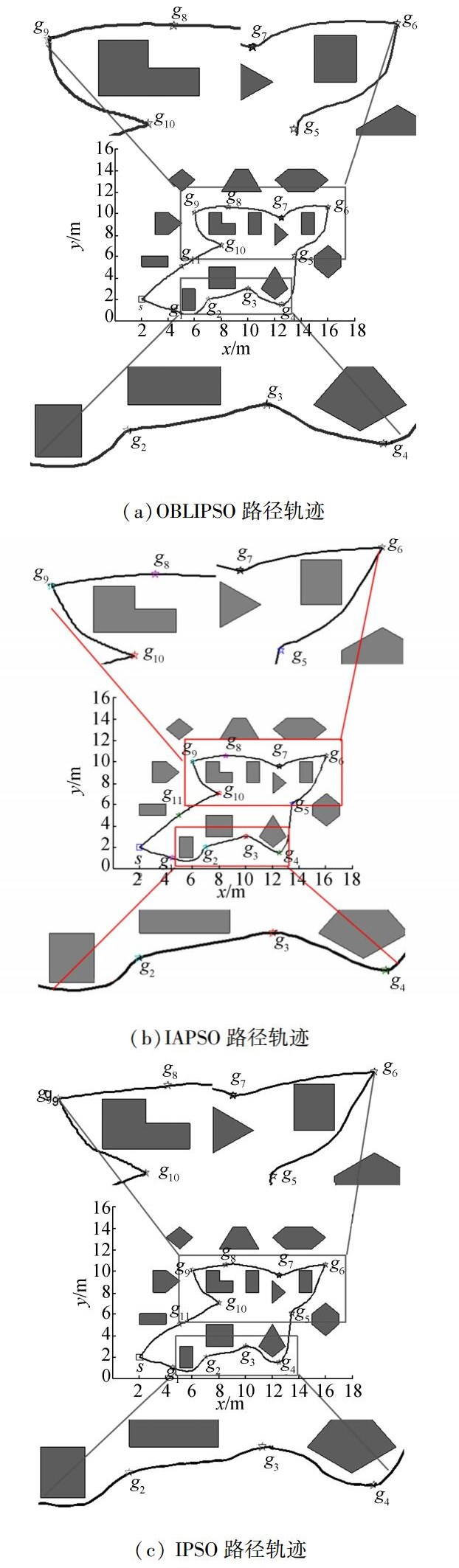图 5 复杂环境下迭代过程 Fig.5 The iteration process in complicated environment表 4 路径性能对比 Tab.4 The performance comparison of path

3) 路径规划性能对比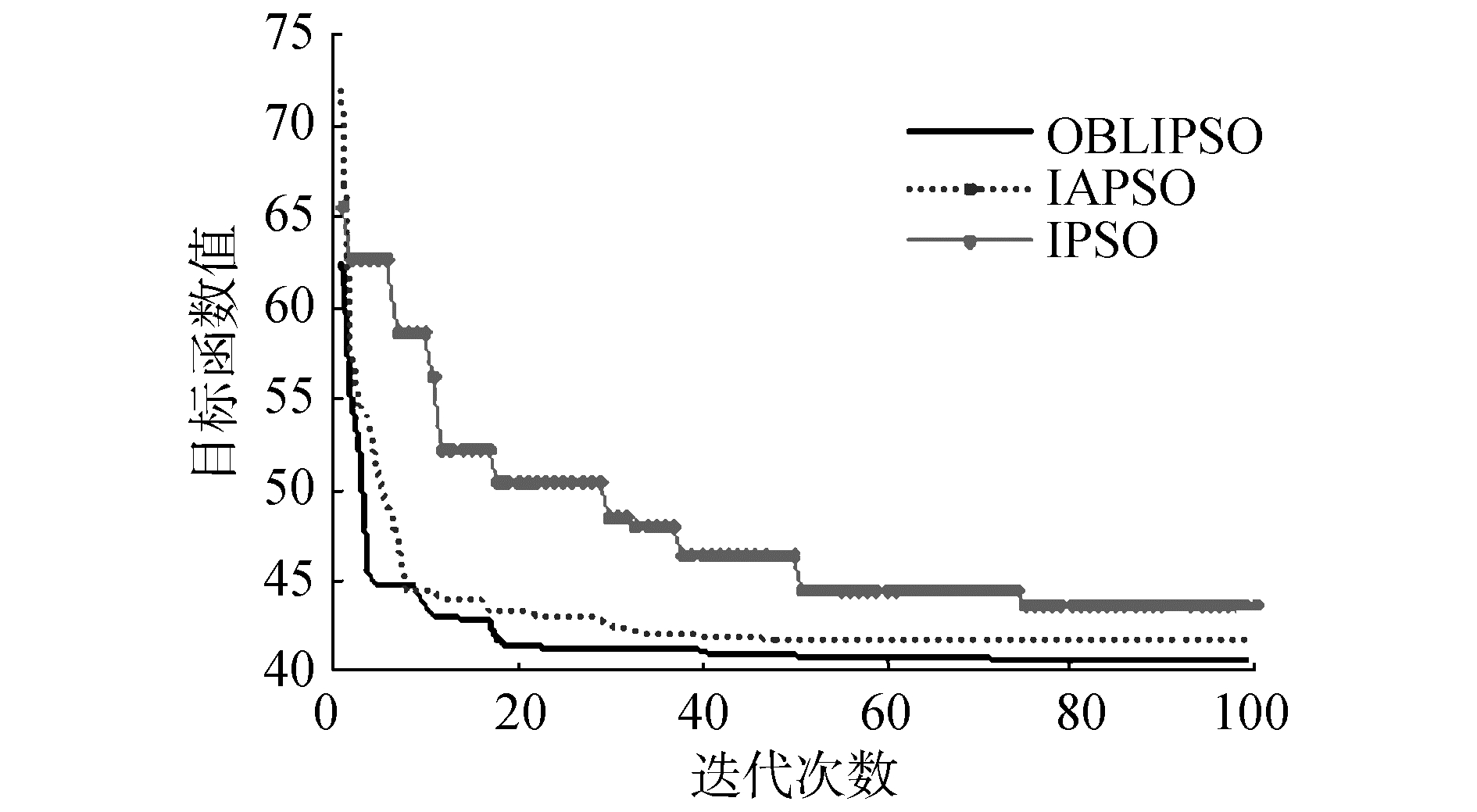图 6 OBLIPSO与IAPSO和IPSO目标函数收敛曲线比较 Fig.6 The convergence comparison of OBLIPSO with IAPSO and IPSO

4.3 实际环境下路径规划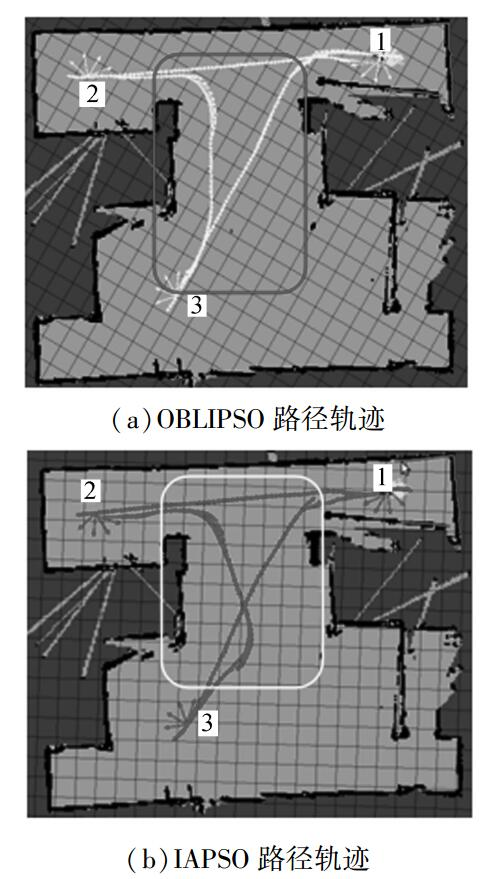图 7 机器人在无障碍环境下的移动过程 Fig.7 The motion process of robot in barrier-freeenvironment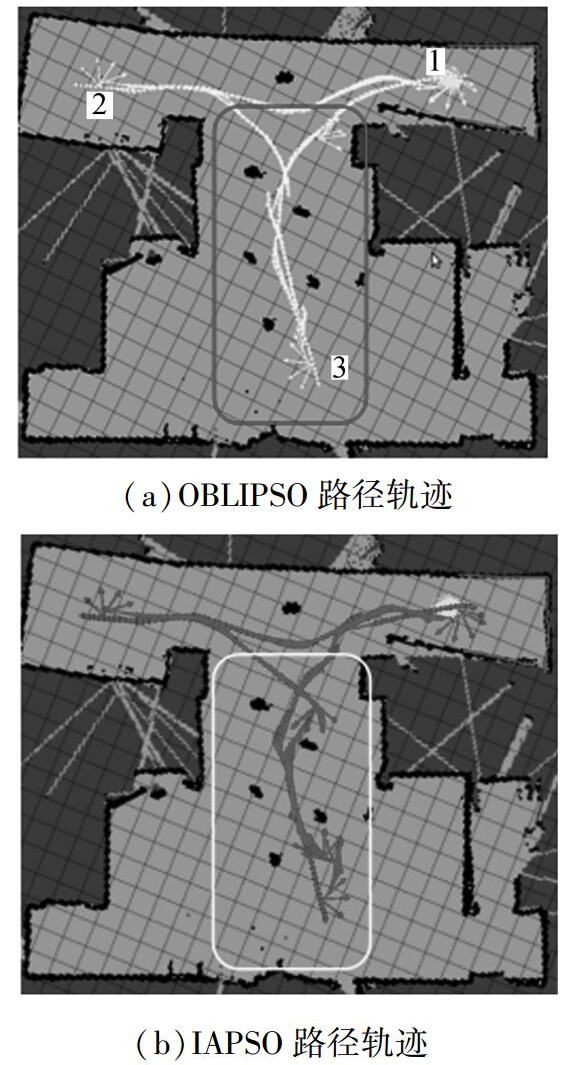图 8 机器人在障碍环境下的移动过程 Fig.8 The motion process of robot in the obstacle environment表 5 真实环境下路径性能对比 Tab.5 The performance comparison of path in real environment
5 结束语

  杨兴, 张亚, 杨巍, 等. 室内移动机器人路径规划研究[J]. 科学技术与工程, 2016, 16(15): 234-238. YANG Xing, ZHANG Ya, YANG Wei, et al. Research on path planning of indoor mobile robot[J]. Science technology and engineering, 2016, 16(15): 234-238. DOI:10.3969/j.issn.1671-1815.2016.15.042 (0)  Ammar A, Bennaceur H, Châari I, et al. Relaxed Dijkstra and A* with linear complexity for robot path planning problems in large-scale grid environments[J]. Soft computing, 2016, 20(10): 4149-4171. DOI:10.1007/s00500-015-1750-1 (0)  杜鹏桢, 唐振民, 孙研. 一种面向对象的多角色蚁群算法及其TSP问题求解[J]. 控制与决策, 2014(10): 1729-1736. DU Pengzhen, TANG Zhenmin, SUN Yan. An object-oriented multi-role ant colony optimization algorithm for solving TSP problem[J]. Control and decision, 2014(10): 1729-1736. (0)  AGRAWAL R K, BAWANE N G. Multiobjective PSO based adaption of neural network topology for pixel classification in satellite imagery[J]. Applied soft computing, 2015, 28(C): 217-225. (0)  DAS P K, BEHERA H S, PANIGRAHI B K. Intelligent-based multi-robot path planning inspired by improved classical Q-learning and improved particle swarm optimization with perturbed velocity[J]. Engineering science and technology, an international journal, 2015, 19(1): 651-669. (0)  YANG Mao, LI Chunzhe. Path planning and tracking for multi-robot system based on improved PSO algorithm[C]//Proceedings of 2011 International Conference on Mechatronic Science, Electric Engineering and Computer(MEC). Jilin: IEEE, 2011: 1667-1670. http://ieeexplore.ieee.org/document/6025799/ (0)  WANG Mingming, LUO Jianjun, WALTER U. Trajectory planning of free-floating space robot using particle swarm optimization(PSO)[J]. Acta astronautica, 2015, 112: 77-88. DOI:10.1016/j.actaastro.2015.03.008 (0)  张万绪, 张向兰, 李莹. 基于改进粒子群算法的智能机器人路径规划[J]. 计算机应用, 2014, 34(2): 510-513. ZHANG Wanxu, ZHANG Xianglan, LI Ying. Path planning for intelligent robots based on improved particle swarm optimization algorithm[J]. Journal of computer applications, 2014, 34(2): 510-513. (0)  王娟, 吴宪祥, 郭宝龙. 基于改进粒子群优化算法的移动机器人路径规划[J]. 计算机工程与应用, 2012, 48(15): 240-244. WANG Juan, WU Xianxiang, GUO Baolong. Robot path planning using improved particle swarm optimization[J]. Computer engineering and applications, 2012, 48(15): 240-244. DOI:10.3778/j.issn.1002-8331.2012.15.049 (0)  张勇, 陈玲, 徐小龙, 等. 基于PSO-GA混合算法时间优化的旅行商问题研究[J]. 计算机应用研究, 2015, 32(12): 3613-3617. ZHANG Yong, CHEN Ling, XU Xiaolong, et al. Research on time optimal TSP based on hybrid PSO-GA[J]. Application research of computers, 2015, 32(12): 3613-3617. DOI:10.3969/j.issn.1001-3695.2015.12.019 (0)  TIZHOOSH H R. Opposition-based learning: a new scheme for machine intelligence[C]//Proceedings of 2005 and International Conference on Intelligent Agents, Web Technologies and Internet Commerce, International Conference on Computational Intelligence for Modelling, Control and Automation. Vienna: IEEE, 2005: 695-701. http://doi.ieeecomputersociety.org/10.1109/CIMCA.2005.1631345 (0)  MUÑOZ D M, LLANOS C H, COELHO L D S, et al. Hardware opposition-based PSO applied to mobile robot controllers[J]. Engineering applications of artificial intelligence, 2014, 28: 64-77. DOI:10.1016/j.engappai.2013.12.003 (0)  汪慎文, 丁立新, 谢大同, 等. 应用反向学习策略的群搜索优化算法[J]. 计算机科学, 2012, 39(9): 183-187. WANG Shenwen, DING Lixin, XIE Datong, et al. Group search optimizer applying opposition-based learning[J]. Computer science, 2012, 39(9): 183-187. (0)  AL-QUNAIEER F S, TIZHOOSH H R, RAHNAMAYAN S. Opposition based computing-a survey[C]//Proceedings of the 2010 International Joint Conference on Neural Networks (IJCNN). Barcelona: IEEE, 2010. http://ieeexplore.ieee.org/xpls/abs_all.jsp?arnumber=5596906 (0)  SURESH K, GHOSH S, KUNDU D, et al. Inertia-adaptive particle swarm optimizer for improved global search[C]//Proceedings of Eighth International Conference on Intelligent Systems Design and Applications. Kaohsiung: IEEE, 2008: 253-258. http://ieeexplore.ieee.org/xpls/icp.jsp?arnumber=4696340 (0)  姜建国, 叶华, 刘慧敏, 等. 融合快速信息交流和局部搜索的粒子群算法[J]. 哈尔滨工程大学学报, 2015, 36(5): 687-691. JIANG Jianguo, YE Hua, LIU Huimin, et al. Particle swarm optimization method with combination of rapid information communication and local search[J]. Journal of Harbin engineering university, 2015, 36(5): 687-691. (0)  GAO Bingkun, REN Xiuju, XU Mingzi. An improved particle swarm algorithm and its application[J]. Procedia engineering, 2011, 15: 2444-2448. DOI:10.1016/j.proeng.2011.08.459 (0)  许少华, 李新幸. 一种自适应改变惯性权重的粒子群算法[J]. 科学技术与工程, 2012, 12(9): 2205-2208. XU Shaohua, LI Xinxing. An adaptive changed inertia weight particle swarm algorithm[J]. Science technology and engineering, 2012, 12(9): 2205-2208. (0)  张建伟, 张立伟, 胡颖, 等. 开源机器人操作系统: ROS[M]. 北京: 科学出版社, 2012. (0)  COUSINS S, GERKEY B, CONLEY K, et al. Sharing Software with ROS[J]. IEEE robotics & automation magazine, 2010, 17(2): 12-14. (0)  ZAMAN S, SLANY W, STEINBAUER G. ROS-based mapping, localization and autonomous navigation using a Pioneer 3-DX robot and their relevant issues[C]//Proceedings of 2011 Saudi International Electronics, Communications and Photonics Conference (SIECPC). Riyadh: IEEE, 2011: 1-5. http://ieeexplore.ieee.org/xpls/icp.jsp?arnumber=5876943 (0)